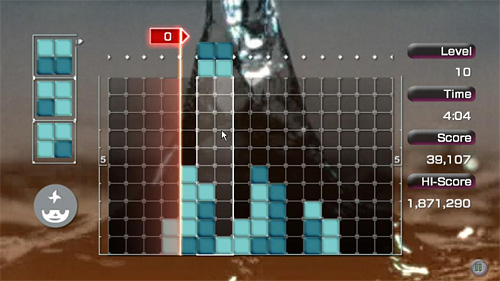# Make a game like Lumines with Flash

I have to admit it… I got completely addicted to this game… so I decided to make my own Flash version.

If you don’t know what is Lumines, it’s a casual game you can download from Steam.The game is simple: control squares made by 2×2 bricks of different colors falling from the top like in Tetris… as you create 2×2 squares in same color, the vertical time line wipes them away from left to right.

In this first part, I’ll create the game field, and the square made by 2×2 bricks, that can be moved and rotated with arrow keys.

There is nothing really hard… but I couldn’t make the entire 2×2 square as a single movieclip because later in the game the square can “break” if it falls over existring bricks.

So every 2×2 square is formed by four movieclips.

There is only one object in the library at the moment… a movieclip linked as `brick` that contains the graphics for the empty grid at frame 1 and the graphics for colored bricks at frames 2-5

Then, I wanted the bricks to move and rotate when the player taps (press and released) arrow keys, not just when he presses them.

The code is completely commented:

```// declaring some setup variables
// number of horizontal cells
grid_width = 16;
// number of vertical cells
grid_height = 10;
// size of the cell
tile_size = 30;
// offset in pixels fron the left side of the stage
x_offset = 10;
// offset in pixels from the top side of the stage
y_offset = 10;
// number of different colors that can be displayed in a brick
different_colors = 3;
// boolean values saying if I should wait for the left (or right, up...) key to be released
// this is used to make the player move bricks tapping arrow keys instead of just pressing them
wait_left = false;
wait_right = false;
wait_up = false;
wait_down = false;
// array containing the game field data
field = new Array();
// array containing the bricks I can move
moveable_bricks = new Array();
// initializing and drawing the play field
for (x=0; x
moveable_bricks[x] = brk.getDepth();
}
// main function, to be executed at every frame
_root.onEnterFrame = function() {
// this is how I detect if a key was tapped:
// when it's pressed, I wait for it to be released (in this case: not pressed)
// thanks to the wait_ variable
if (Key.isDown(Key.LEFT)) {
wait_left = true;
} else {
if (wait_left) {
// if the left key has been tapped, move the four bricks on the left
for (x=0; x<4; x++) {
// this "if" is used to determine if the bricks are still inside the game field
if ((_root["brick_"+moveable_bricks[x]]._x-x_offset)/tile_size-_root["brick_"+moveable_bricks[x]].pos%2>0) {
_root["brick_"+moveable_bricks[x]]._x -= tile_size;
}
}
// reset variable, now I must wait again for a key to be pressed
wait_left = false;
}
}
// same routine for the right key
if (Key.isDown(Key.RIGHT)) {
wait_right = true;
} else {
if (wait_right) {
for (x=0; x<=3; x++) {
if ((_root["brick_"+moveable_bricks[x]]._x-x_offset)/tile_size-_root["brick_"+moveable_bricks[x]].pos%2<14) {
_root["brick_"+moveable_bricks[x]]._x += tile_size;
}
}
wait_right = false;
}
}
// when the DOWN arrow is pressed, I must rotate the bricks clockwise
// block 0: moves to the right and becomes block 1
// block 1: moves down and becomes block 3
// block 2: moves up and becomes block 0
// block 3: moves to the left and becomes block 2
if (Key.isDown(Key.DOWN)) {
wait_down = true;
} else {
if (wait_down) {
for (x=0; x<4; x++) {
switch (_root["brick_"+moveable_bricks[x]].pos) {
case 0 :
_root["brick_"+moveable_bricks[x]].pos = 1;
_root["brick_"+moveable_bricks[x]]._x += tile_size;
break;
case 1 :
_root["brick_"+moveable_bricks[x]].pos = 3;
_root["brick_"+moveable_bricks[x]]._y += tile_size;
break;
case 2 :
_root["brick_"+moveable_bricks[x]].pos = 0;
_root["brick_"+moveable_bricks[x]]._y -= tile_size;
break;
case 3 :
_root["brick_"+moveable_bricks[x]].pos = 2;
_root["brick_"+moveable_bricks[x]]._x -= tile_size;
break;
}
}
wait_down = false;
}
}
// when the UP arrow is pressed, I must rotate the bricks counter-clockwise
// block 0: moves down the right and becomes block 2
// block 1: moves to the left and becomes block 0
// block 2: moves to the right and becomes block 3
// block 3: moves up and becomes block 1
if (Key.isDown(Key.UP)) {
wait_up = true;
} else {
if (wait_up) {
for (x=0; x<4; x++) {
switch (_root["brick_"+moveable_bricks[x]].pos) {
case 0 :
_root["brick_"+moveable_bricks[x]].pos = 2;
_root["brick_"+moveable_bricks[x]]._y += tile_size;
break;
case 1 :
_root["brick_"+moveable_bricks[x]].pos = 0;
_root["brick_"+moveable_bricks[x]]._x -= tile_size;
break;
case 2 :
_root["brick_"+moveable_bricks[x]].pos = 3;
_root["brick_"+moveable_bricks[x]]._x += tile_size;
break;
case 3 :
_root["brick_"+moveable_bricks[x]].pos = 1;
_root["brick_"+moveable_bricks[x]]._y -= tile_size;
break;
}
}
wait_up = false;
}
}
};```

and here it is the result: move and rotate the square with arrow keys.

here it is the source code for you to experiment.

214 GAME PROTOTYPES EXPLAINED WITH SOURCE CODE
// 1+2=3
// 10000000
// 2 Cars
// 2048
// Avoider
// Ballz
// Block it
// Blockage
// Bloons
// Boids
// Bombuzal
// Breakout
// Bricks
// Columns
// CubesOut
// Dots
// DROP'd
// Dudeski
// Eskiv
// Filler
// Fling
// Globe
// HookPod
// Hundreds
// InkTd
// Iromeku
// Lumines
// Magick
// MagOrMin
// Maze
// Memdot
// Nano War
// Nodes
// o:anquan
// Ononmin
// Pacco
// Phyballs
// Platform
// Poker
// Pool
// Poux
// Pudi
// qomp
// Racing
// Renju
// SameGame
// Security
// Sling
// Slingy
// Sokoban
// Splitter
// Sproing
// Stack
// Stringy
// Sudoku
// Tetris
// Threes
// Toony
// Turn
// TwinSpin
// vvvvvv
// Wordle
// Worms
// Yanga
// Zhed
// zNumbers